## Solutions to Try Its

1. The equation fails the symmetry test with respect to the line $\theta =\frac{\pi }{2}$ and with respect to the pole. It passes the polar axis symmetry test.

2. Tests will reveal symmetry about the polar axis. The zero is $\left(0,\frac{\pi }{2}\right)$, and the maximum value is $\left(3,0\right)$.

3.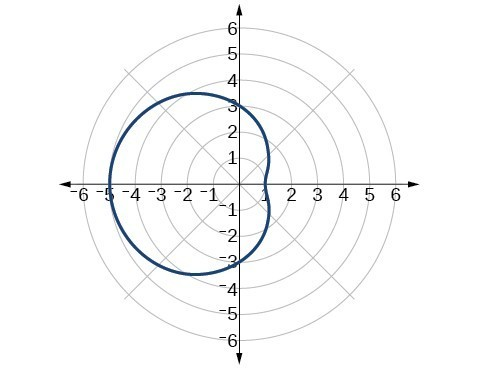4. The graph is a rose curve, $n$ even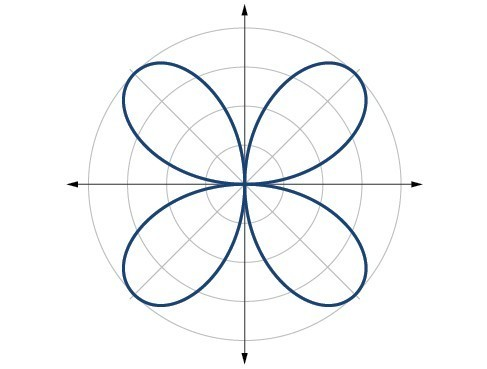5. Rose curve, $n$ odd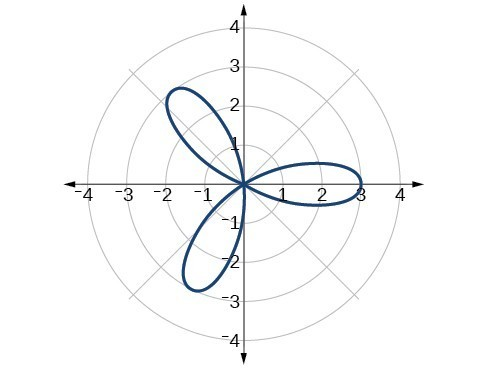6.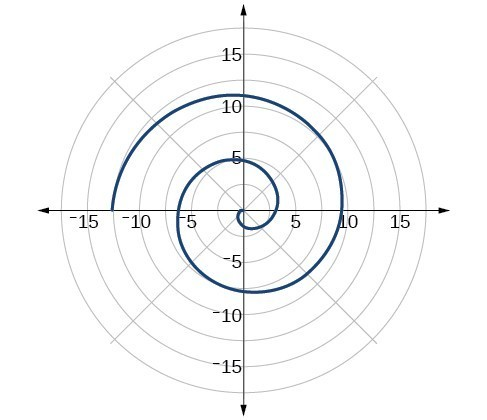## Solutions to Odd-Numbered Exercises

1. Symmetry with respect to the polar axis is similar to symmetry about the $x$ -axis, symmetry with respect to the pole is similar to symmetry about the origin, and symmetric with respect to the line $\theta =\frac{\pi }{2}$ is similar to symmetry about the $y$ -axis.

3. Test for symmetry; find zeros, intercepts, and maxima; make a table of values. Decide the general type of graph, cardioid, limaçon, lemniscate, etc., then plot points at $\theta =0,\frac{\pi }{2},\pi \text{and }\frac{3\pi }{2}$, and sketch the graph.

5. The shape of the polar graph is determined by whether or not it includes a sine, a cosine, and constants in the equation.

7. symmetric with respect to the polar axis

9. symmetric with respect to the polar axis, symmetric with respect to the line $\theta =\frac{\pi }{2}$, symmetric with respect to the pole

11. no symmetry

13. no symmetry

15. symmetric with respect to the pole

17. circle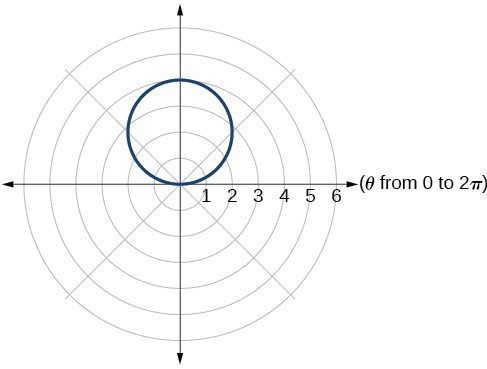19. cardioid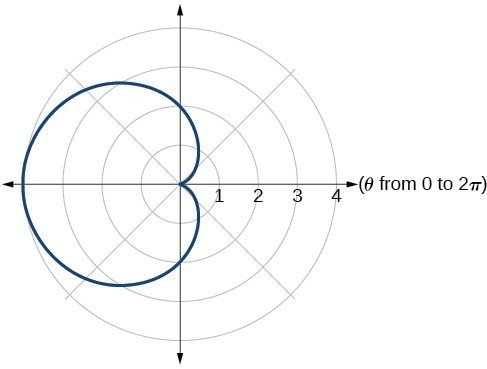21. cardioid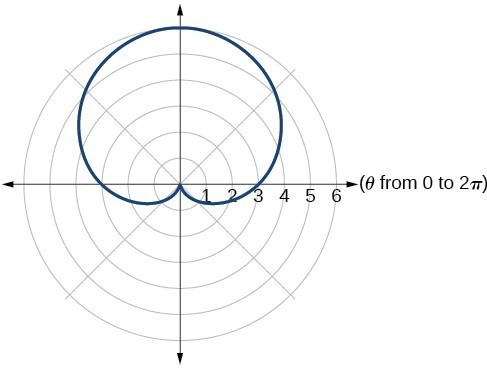23. one-loop/dimpled limaçon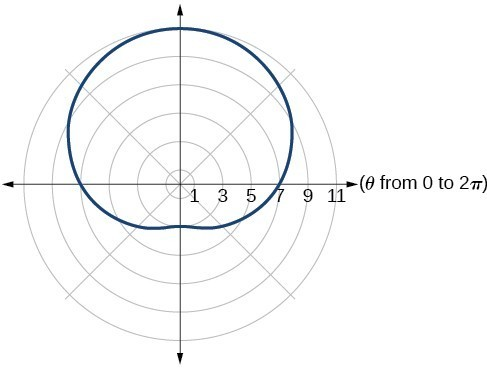25. one-loop/dimpled limaçon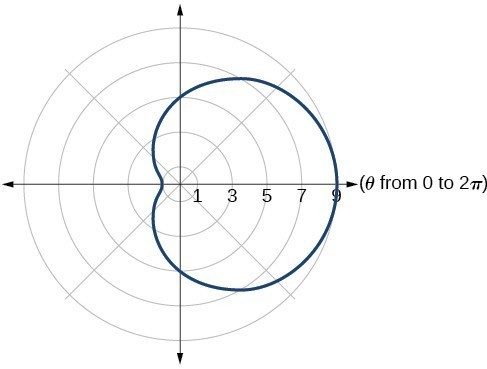27. inner loop/two-loop limaçon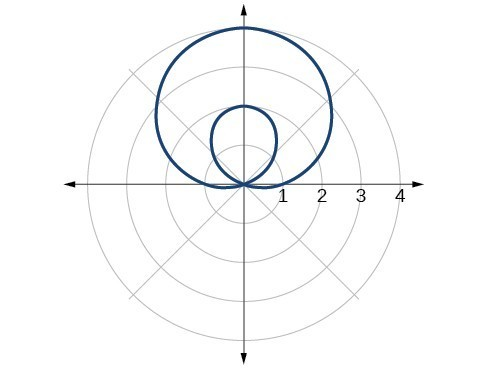29. inner loop/two-loop limaçon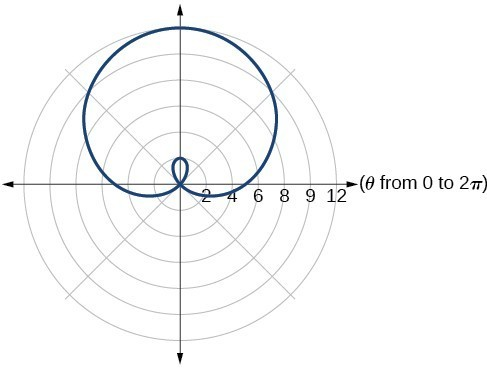31. inner loop/two-loop limaçon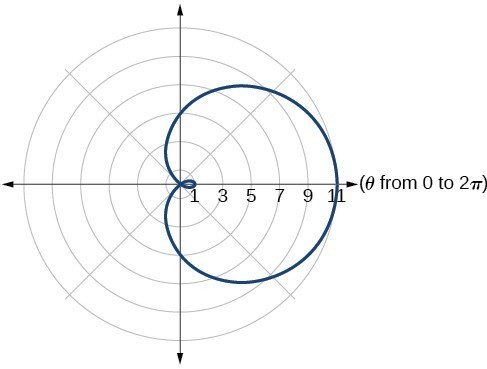33. lemniscate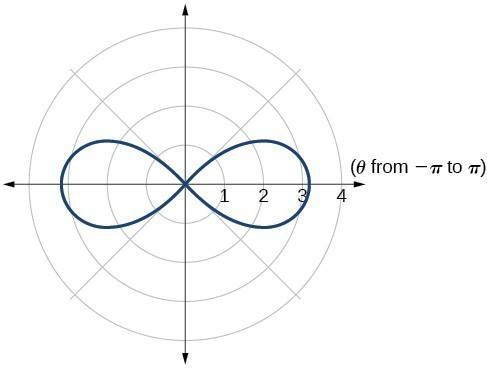35. lemniscate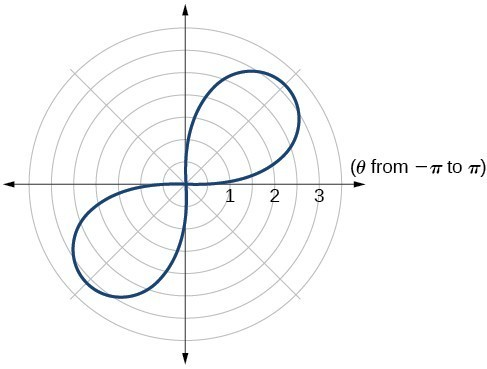37. rose curve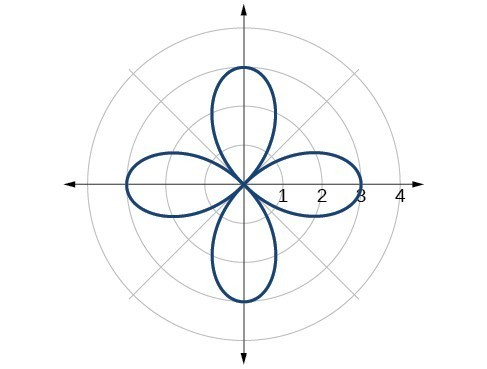39. rose curve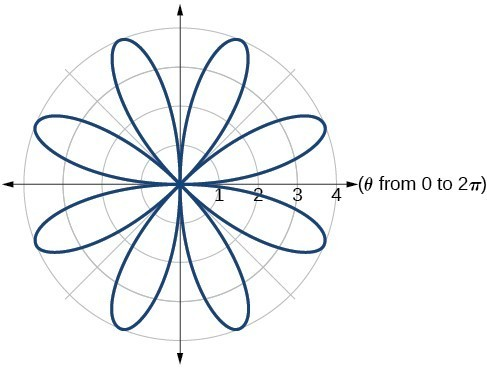41. Archimedes’ spiral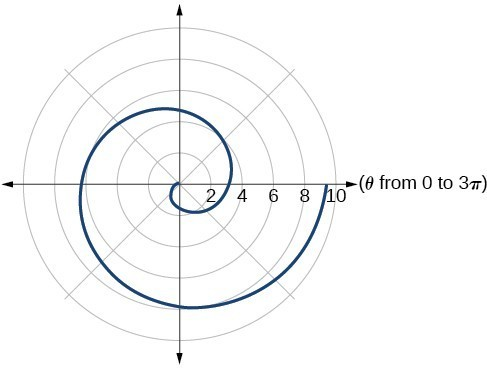43. Archimedes’ spiral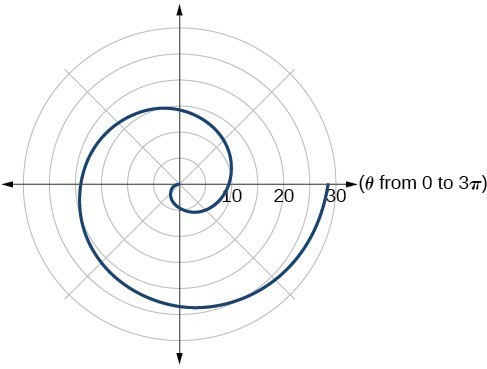45.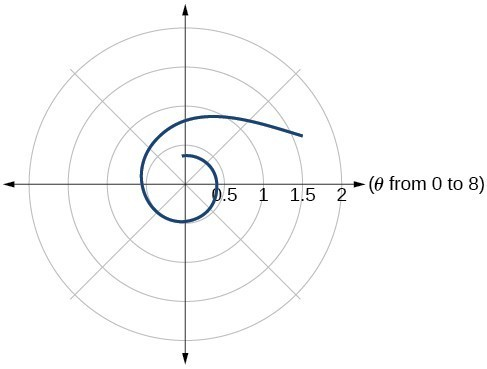47.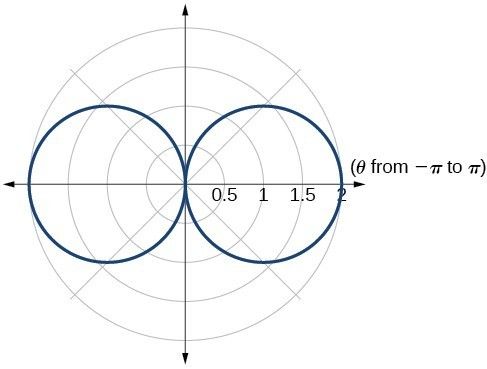49.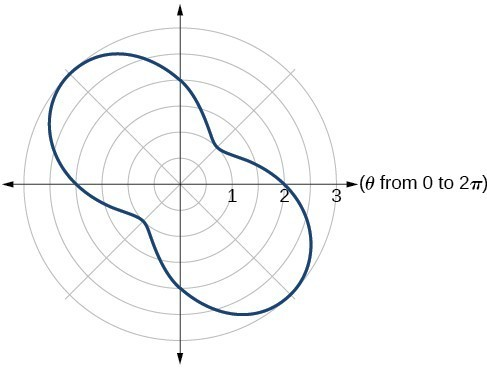51.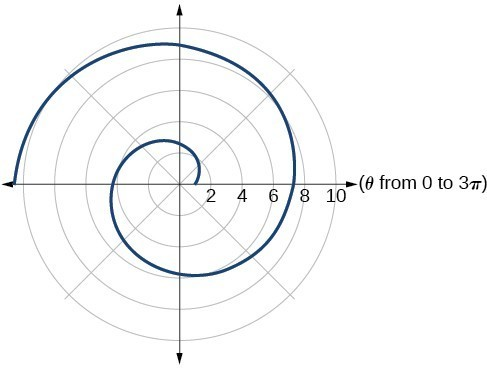53.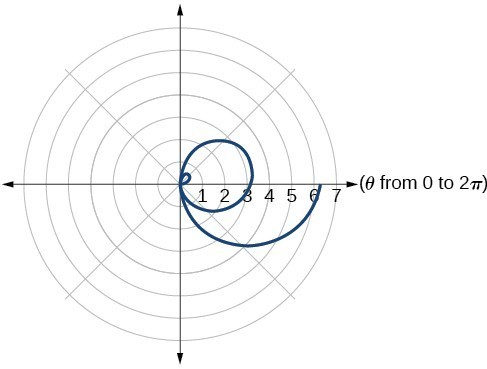55. They are both spirals, but not quite the same.

57. Both graphs are curves with 2 loops. The equation with a coefficient of $\theta$ has two loops on the left, the equation with a coefficient of 2 has two loops side by side. Graph these from 0 to $4\pi$ to get a better picture.

59. When the width of the domain is increased, more petals of the flower are visible.

61. The graphs are three-petal, rose curves. The larger the coefficient, the greater the curve’s distance from the pole.

63. The graphs are spirals. The smaller the coefficient, the tighter the spiral.

65. $\left(4,\frac{\pi }{3}\right),\left(4,\frac{5\pi }{3}\right)$

67. $\left(\frac{3}{2},\frac{\pi }{3}\right),\left(\frac{3}{2},\frac{5\pi }{3}\right)$

69. $\left(0,\frac{\pi }{2}\right),\left(0,\pi \right),\left(0,\frac{3\pi }{2}\right),\left(0,2\pi \right)$

71. $\left(\frac{\sqrt{8}}{2},\frac{\pi }{4}\right),\left(\frac{\sqrt{8}}{2},\frac{5\pi }{4}\right)$
and at $\theta =\frac{3\pi }{4},\frac{7\pi }{4}$ since $r$ is squared